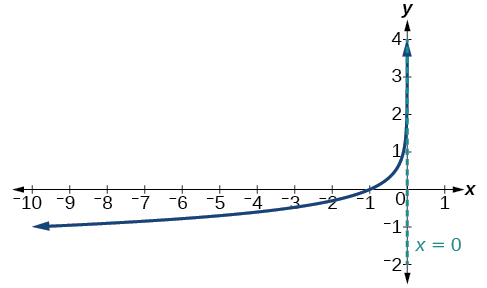6.4 Graphs of logarithmic functions  (Page 6/8)

 Page 6 / 8

Given a logarithmic function with the parent function $\text{\hspace{0.17em}}f\left(x\right)={\mathrm{log}}_{b}\left(x\right),$ graph a translation.

1. Draw the vertical asymptote, $\text{\hspace{0.17em}}x=0.$
1. Draw the vertical asymptote, $\text{\hspace{0.17em}}x=0.$
1. Plot the x- intercept, $\text{\hspace{0.17em}}\left(1,0\right).$
1. Plot the x- intercept, $\text{\hspace{0.17em}}\left(1,0\right).$
1. Reflect the graph of the parent function $\text{\hspace{0.17em}}f\left(x\right)={\mathrm{log}}_{b}\left(x\right)\text{\hspace{0.17em}}$ about the x -axis.
1. Reflect the graph of the parent function $\text{\hspace{0.17em}}f\left(x\right)={\mathrm{log}}_{b}\left(x\right)\text{\hspace{0.17em}}$ about the y -axis.
1. Draw a smooth curve through the points.
1. Draw a smooth curve through the points.
1. State the domain, $\text{\hspace{0.17em}}\left(0,\infty \right),$ the range, $\text{\hspace{0.17em}}\left(-\infty ,\infty \right),$ and the vertical asymptote $\text{\hspace{0.17em}}x=0.$
1. State the domain, $\text{\hspace{0.17em}}\left(-\infty ,0\right),$ the range, $\text{\hspace{0.17em}}\left(-\infty ,\infty \right),$ and the vertical asymptote $\text{\hspace{0.17em}}x=0.$

Graphing a reflection of a logarithmic function

Sketch a graph of $\text{\hspace{0.17em}}f\left(x\right)=\mathrm{log}\left(-x\right)\text{\hspace{0.17em}}$ alongside its parent function. Include the key points and asymptote on the graph. State the domain, range, and asymptote.

Before graphing $\text{\hspace{0.17em}}f\left(x\right)=\mathrm{log}\left(-x\right),$ identify the behavior and key points for the graph.

• Since $\text{\hspace{0.17em}}b=10\text{\hspace{0.17em}}$ is greater than one, we know that the parent function is increasing. Since the input value is multiplied by $\text{\hspace{0.17em}}-1,$ $f\text{\hspace{0.17em}}$ is a reflection of the parent graph about the y- axis. Thus, $\text{\hspace{0.17em}}f\left(x\right)=\mathrm{log}\left(-x\right)\text{\hspace{0.17em}}$ will be decreasing as $\text{\hspace{0.17em}}x\text{\hspace{0.17em}}$ moves from negative infinity to zero, and the right tail of the graph will approach the vertical asymptote $\text{\hspace{0.17em}}x=0.\text{\hspace{0.17em}}$
• The x -intercept is $\text{\hspace{0.17em}}\left(-1,0\right).$
• We draw and label the asymptote, plot and label the points, and draw a smooth curve through the points.

The domain is $\text{\hspace{0.17em}}\left(-\infty ,0\right),$ the range is $\text{\hspace{0.17em}}\left(-\infty ,\infty \right),$ and the vertical asymptote is $\text{\hspace{0.17em}}x=0.$

Graph $\text{\hspace{0.17em}}f\left(x\right)=-\mathrm{log}\left(-x\right).\text{\hspace{0.17em}}$ State the domain, range, and asymptote.The domain is $\text{\hspace{0.17em}}\left(-\infty ,0\right),$ the range is $\text{\hspace{0.17em}}\left(-\infty ,\infty \right),$ and the vertical asymptote is $\text{\hspace{0.17em}}x=0.$

Given a logarithmic equation, use a graphing calculator to approximate solutions.

1. Press [Y=] . Enter the given logarithm equation or equations as Y 1 = and, if needed, Y 2 = .
2. Press [GRAPH] to observe the graphs of the curves and use [WINDOW] to find an appropriate view of the graphs, including their point(s) of intersection.
3. To find the value of $\text{\hspace{0.17em}}x,$ we compute the point of intersection. Press [2ND] then [CALC] . Select “intersect” and press [ENTER] three times. The point of intersection gives the value of $\text{\hspace{0.17em}}x,$ for the point(s) of intersection.

Approximating the solution of a logarithmic equation

Solve $\text{\hspace{0.17em}}4\mathrm{ln}\left(x\right)+1=-2\mathrm{ln}\left(x-1\right)\text{\hspace{0.17em}}$ graphically. Round to the nearest thousandth.

Press [Y=] and enter $\text{\hspace{0.17em}}4\mathrm{ln}\left(x\right)+1\text{\hspace{0.17em}}$ next to Y 1 =. Then enter $\text{\hspace{0.17em}}-2\mathrm{ln}\left(x-1\right)\text{\hspace{0.17em}}$ next to Y 2 = . For a window, use the values 0 to 5 for $\text{\hspace{0.17em}}x\text{\hspace{0.17em}}$ and –10 to 10 for $\text{\hspace{0.17em}}y.\text{\hspace{0.17em}}$ Press [GRAPH] . The graphs should intersect somewhere a little to right of $\text{\hspace{0.17em}}x=1.$

For a better approximation, press [2ND] then [CALC] . Select [5: intersect] and press [ENTER] three times. The x -coordinate of the point of intersection is displayed as 1.3385297. (Your answer may be different if you use a different window or use a different value for Guess? ) So, to the nearest thousandth, $\text{\hspace{0.17em}}x\approx 1.339.$

Solve $\text{\hspace{0.17em}}5\mathrm{log}\left(x+2\right)=4-\mathrm{log}\left(x\right)\text{\hspace{0.17em}}$ graphically. Round to the nearest thousandth.

$x\approx 3.049$

Summarizing translations of the logarithmic function

Now that we have worked with each type of translation for the logarithmic function, we can summarize each in [link] to arrive at the general equation for translating exponential functions.

A laser rangefinder is locked on a comet approaching Earth. The distance g(x), in kilometers, of the comet after x days, for x in the interval 0 to 30 days, is given by g(x)=250,000csc(π30x). Graph g(x) on the interval [0, 35]. Evaluate g(5)  and interpret the information. What is the minimum distance between the comet and Earth? When does this occur? To which constant in the equation does this correspond? Find and discuss the meaning of any vertical asymptotes.
The sequence is {1,-1,1-1.....} has
how can we solve this problem
Sin(A+B) = sinBcosA+cosBsinA
Prove it
Eseka
Eseka
hi
Joel
June needs 45 gallons of punch. 2 different coolers. Bigger cooler is 5 times as large as smaller cooler. How many gallons in each cooler?
7.5 and 37.5
Nando
find the sum of 28th term of the AP 3+10+17+---------
I think you should say "28 terms" instead of "28th term"
Vedant
the 28th term is 175
Nando
192
Kenneth
if sequence sn is a such that sn>0 for all n and lim sn=0than prove that lim (s1 s2............ sn) ke hole power n =n
write down the polynomial function with root 1/3,2,-3 with solution
if A and B are subspaces of V prove that (A+B)/B=A/(A-B)
write down the value of each of the following in surd form a)cos(-65°) b)sin(-180°)c)tan(225°)d)tan(135°)
Prove that (sinA/1-cosA - 1-cosA/sinA) (cosA/1-sinA - 1-sinA/cosA) = 4
what is the answer to dividing negative index
In a triangle ABC prove that. (b+c)cosA+(c+a)cosB+(a+b)cisC=a+b+c.
give me the waec 2019 questionsByByBy Lakeima RobertsBy Anindyo MukhopadhyayBy